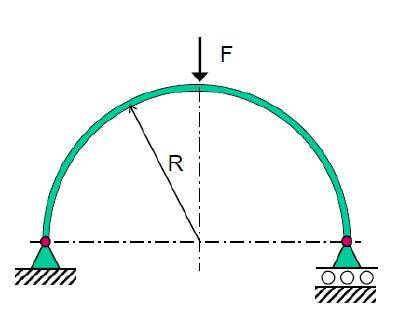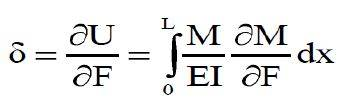# Castigliano's Second Theorem on Curved Structures

## Homework Statement

A semi-circular ring of stiffness EI and radius R is supported on an anchored hinge and on a roller hinge. A vertical load F is applied at the center. Determine the horizontal displacement of the roller support.## Homework Equations## The Attempt at a Solution

So I apply a horizontal force H (to the right) at the roller. Sectioning the first quadrant gives $$M_1=HR sinθ_1$$ (moment is taken as positive clockwise). So $$\frac{∂M_1}{∂H}=R sinθ_1$$. Similarly, $$M_2=HR cosθ_2-FRsinθ_2$$ and $$\frac{∂M_2}{∂H}=R cosθ_2$$. Because H is an imaginary load and setting it to 0, $$M_1=0$$, $$\frac{∂M_1}{∂H}=R sinθ_1$$, $$M_2=-FRsinθ_2$$ and $$\frac{∂M_2}{∂H}=R cosθ_2$$. So the integral is $$\int_0^ \frac{π}{2} \frac{M_1}{EI} \frac{∂M_1}{∂H}R dθ_1 + \int_0^ \frac{π}{2} \frac{M_2}{EI} \frac{∂M_2}{∂H}R dθ_2$$. The first integral is zero. The second gives $$\int_0^ \frac{π}{2} \frac{-FR^3}{2EI} sin2θ_2 dθ_2=\frac{-FR^3}{2EI}$$. I checked my workings, and I have no idea why there is a negative sign there. If my understanding is correct, the negative sign implies roller movement to the left, and this is really counter intuitive. Can anyone shed some light on this?